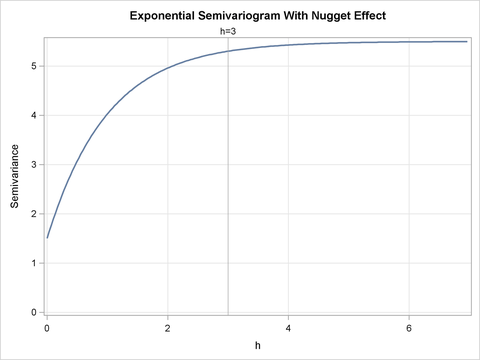The Nugget Effect

For all the semivariogram models considered previously, the following property holds: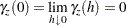However, a plot of the experimental semivariogram might indicate a discontinuity at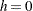; that is,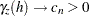as
, while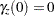. The quantity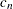is called the nugget effect; this term is from mining geostatistics where nuggets literally exist, and it represents variations at a much smaller scale than any of the measured pairwise distances—that is, at distances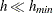, where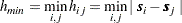Nonzero nugget effects have been associated with conceptual and theoretical difficulties; see Cressie (1993, section 2.3.1) and Christakos (1992, section 7.4.3) for details. There is no practical difficulty, however; you simply visually extrapolate the experimental semivariogram as. The importance of availability of data at small lag distances is again illustrated.

As an example, an exponential semivariogram with a nugget effecthas the form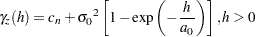and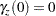where the factor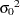is called the partial sill and the sill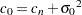.

This is illustrated in Figure 48.11 for the parameters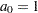,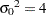, and nugget effect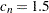.

You can specify the nugget effect in PROC KRIGE2D with the NUGGET= option in the MODEL statement. It is a separate, additive term independent of direction; that is, it is isotropic. The way to approximate an anisotropic nugget effect is described in the following section.

Figure 48.11 Exponential Semivariogram Model with a Nugget Effect